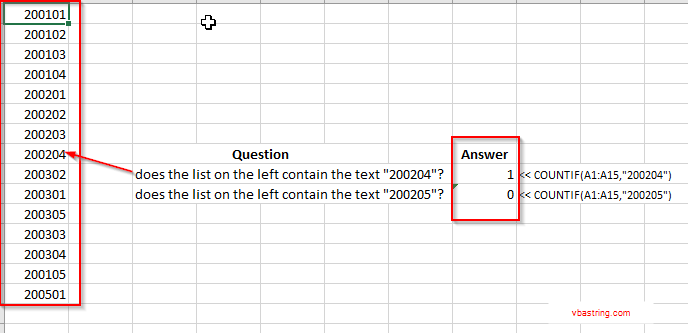# How To Have Excel Check If Range Of Cells Contains Specific Text With VBA

Here’s an example of how to check if a value exists in a range of cells:

It is actually a response from a question I received:

“Can you let me know how to check if a particular value is present in a named list. Or in a different worksheet”

You can use the “countif” function.

ex. =countif({value to look for}, {range to look in})

if the result is greater than zero, the value is in the range, if zero then it’s not there.Take a look at the above example.

You’ll see that the built in countif Excel function returns a value greater than zero if the value be sought after is in the list (range), and zero if it’s not.

## Now how do you use the countif function with VBA?

* Here I’m using the “countif” function, and returning the value to a variable called “intct” :

intct = Application.WorksheetFunction.CountIf(Range(“F1:F” & lngLastRow), strInvoiceID)

Questions? Contact Me